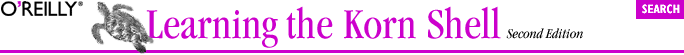home | O'Reilly's CD bookshelfs | FreeBSD | Linux | Cisco | Cisco Exam## 6.3. Arithmetic for

The for loop as described in Chapter 5 has been in Unix shells since the Version 7 Bourne Shell. As mentioned, you can't do Pascal or C-style looping for a fixed number of iterations with that for loop. ksh93 introduced the arithmetic for loop to remedy this situation and to bring the shell closer to a traditional (some would say "real") programming language.

The syntax resembles the shell's arithmetic facilities that we have just seen. It is almost identical to the syntax of the C for loop, except for the extra set of parentheses:

```for ((init; condition; increment))
do
statements ...
done
```

Here, init represents something to be done once, at the start of the loop. The condition is tested, and as long as it's true, the shell executes statements. Before going back to the top of the loop to test the condition again, the shell executes increment.

Any of init, condition, and increment may be omitted. A missing condition is treated as true; i.e., the loop body always executes. (The so-called "infinite loop" requires you to use some other method to leave the loop.) We'll use the arithmetic for loop for Task 6-2, which is our next, rather simple task.

Here's the code; the explanation follows:

```sum=0
count=\$#
for ((i = 1; i <= count; i++))
do
let "sum += \$1"
shift
done
print \$sum
```

The first line initializes the variable sum to 0. sum accumulates the sum of the arguments. The second line is mostly for readability; count indicates how many arguments there are. The third line starts the loop itself. The variable i is the loop control variable. The init clause sets it to 1, the condition clause tests it against the limit count, and the increment clause adds 1 to it each time around the loop. One thing you'll notice right away is that inside the for loop header, there's no need for the \$ in front of a variable name to get that variable's value. This is true for any arithmetic expression in the Korn shell.

The body of the loop does the addition. It simply lets let do the math: the addition is accomplished by adding \$1 to the value in sum. The shift command then moves the next argument down into \$1 for use the next time around the loop. Finally, when the loop is done, the script prints the result.

The arithmetic for loop is particularly handy for working with all the elements in an indexed array, which we're about to see in the next section.